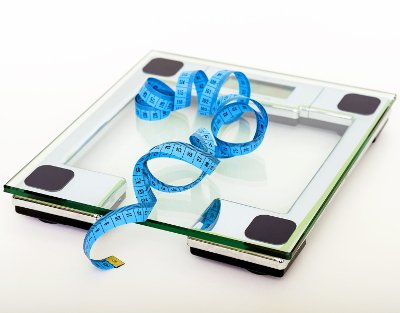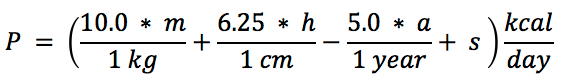# Weight Loss Calculator / Lose Weight Calculator

Share:

Do you want to know how long it will take to lose weight to reach your target weight? Or How much calories you have to burn every day to reach your desired weight? Here is the weight loss calculator for you.

Please scroll down for details on using this weight loss calculator.

*
*
*
ft
Feet
in
Inches
cm
Centimeters
*
lb
Pounds
kg
Kilograms
*
lb
Pounds
kg
Kilograms

#### Choose one among the below fields and enter value.

lb
Pounds
kg
Kilograms

Result:

Please enter the details above and click Calculate button to get your weight loss result.

Similar Fitness Calculators

## Weight Loss CalculatorThis body weight loss calculator is for those who have the below questions:

1. How many calories do I need to burn every day to achieve my desired weight?
2. How long will it take to reduce my weight and reach my desired target weight?
3. When will I reach my desired dream weight?
4. How do I calculate my target calorie intake to reduce my weight?

This body weight loss goal calculator is based on the Mifflin St Jeor Basal Metabolic Rate (BMR) Equation. It takes your gender, height, current weight, desired weight and calculates your weight loss goal in the form of target calorie intake per day. It also provides you how long it will take to lose your weight and reach your goal weight. You will also have the option to select your desired calorie deficit to reach your target weight. This acts as a calorie loss calculator to identify the calorie deficit needed to achieve your target weight at the target date.

### Using the Weight Loss calculator

3. Enter your Height either in centimeters or in feet.
4. Enter your Current Weight either in kilograms or in pounds.
5. Enter your Target Weight either in kilograms or in pounds.
7. Enter one among the below options:
1. Enter the Target Weight Loss Per Week either in kilograms or in pounds.
2. (or) Select the Target Calorie Deficit Per Day.
3. (or) Select the Target Date to achieve your goal weight.
8. Then, click the Calculate button. The daily calorie needs to lose weight will be displayed in the result section

### Conditions and limitations

1. For women, it is dangerous to reduce the calorie intake below 1200 calories/day. So, this calculator won't allow the calorie loss below this limit
2. For men, it is dangerous to reduce the calorie intake below 1500 calories/day. So, this calculator won't allow the calorie loss below this limit
3. Recommended weight loss per week is ½ lb to 2 lb (approximately ¼ Kg to 1 Kg)
4. This weight loss calculation is just an estimate to give you an idea, for accurate weight loss goals contact professional health advisors.

## Weight Loss Formulas

Weight loss goal calculation is based on basal metabolic rate (BMR) Equation.

### Basal Metabolic Rate (BMR) Formula

BMR can be calculated using several formulas. among them Mifflin St Jeor Equation is considered as the most accurate and reliable. This body weight loss calculator uses Mifflin St Jeor Equation for calculating BMR and then multiplies the BMR with the Activity Factor to find the energy needed by the human body every day. Read more about BMR here.

#### The Mifflin St Jeor EquationWhere:

• P = Total energy consumed at rest in kcal per day.
• s = A constant. Substitute +5 for men and -161 for women.
• m = Mass in kilogram (kg).
• h = Height in centimeters (cm).
• a = Age in years.

### Activity Factor

Activity factor is determined by the lifestyle of an individual. Activity factor divides the physical lifestyle of a person into multiple categories. Every category is represented with a numeric factor. multiplying the numeric factor with the calculated BMR will give the daily calorie needed by a person.

Activity Category Description Activity Factor
SedentaryAn office worker, who do not have any option to move around and won't do any exercise.1.2
Lightly ActiveAn office worker, who moves around or will do little exercise every day. (or) Exercising 1 to 3 days per week.1.375
Moderately ActivePerson who works by standing or doing moderate exercises like running or swimming for an hour every day. (or) Exercising 3 to 5 days per week.1.55
Vigorously ActiveHighly active person or doing exercises like swimming or running for two hours every day. (or) Exercising 6 to 7 days per week.1.725
Extremely ActiveAthletes or sports person spending lot of time in a day practicing and doing exercise.1.9

## How to Lose Weight

Weight loss can be achieved by reducing the food intake or burn more calories or combining both so that the calorie balance per day should be less than the daily calorie needed to maintain the current weight. Below is an example for calorie reduction options.

### Example

Consider a person's daily calorie needed to maintain the weight is 2200 Cal/day. For reducing the weight the calorie needs to be reduced to something like 1800 Cal/day. The question is how to maintain a lower calorie intake per day. There are several ways to achieve a lower calorie intake. They are:

#### Reducing the net calorie intake to lose weight

1. Do more exercise than usual and burn 400 calories so as the net calorie intake per day is 1800.
2. Eat less than normal food and bring down the calorie intake to 1800 Cal/day.
3. Use the combination of above and make sure the daily calorie intake is 2000 Cal/day and do exercise and burn 200 Cal/day, so as the net calorie intake is 1800 Cal/day